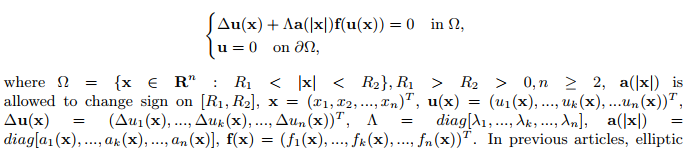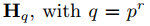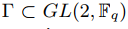##### New Criterion of Robust Hܣ Stabilization for Uncertain Neutral Systems

Jiyong Lu

Asian Research Journal of Mathematics, Page 1-12
DOI: 10.9734/arjom/2021/v17i630304

The problems of delay-dependent robust stability and stabilization for a class of uncertain neutral systems are investigated in this paper. At first, by constructing a new Lyapunov functional and using the Lyapunov stability theory, a new delay-dependent condition which renders the system with no external disturbance and input to be asymptotically stable is obtained and given by a linear matrix inequality. Then, based on the obtained condition, a state feedback stabilize law is designed, which guarantees closed-loop neutral systems are asymptotically stable for all the permitted uncertainties when the external disturbance is naught, and it can also guarantee the closed-loop systems have  performance under the external disturbance. The model of neutral systems with both the uncertainty and the disturbance discussed in this paper has rarely been considered before.

##### More on Symmetric Brothers of a Node in a Perfect Binary Tree

Xingbo Wang

Asian Research Journal of Mathematics, Page 13-26
DOI: 10.9734/arjom/2021/v17i630305

The paper makes an extensive study on the symmetric brothers of a node in a perfect binary tree. Through proving several new properties of the symmetric brothers of a node, it reveals how the symmetric brothers and the symmetric ancestors distribute on the tree and how they are beneficial for designing a searching algorithm of special purpose. Detail mathematical reasoning and proofs are shown together with concrete examples to demonstrate the mathematical traits. The paper is helpful for designing algorithms in blind search related aspects.

##### Other Families of Rational Solutions to the KPI Equation

Pierre Gaillard

Asian Research Journal of Mathematics, Page 27-34
DOI: 10.9734/arjom/2021/v17i630306

Aims / Objectives: We present rational solutions to the Kadomtsev-Petviashvili equation (KPI) in terms of polynomials in x, y and t depending on several real parameters. We get an infinite hierarchy of rational solutions written as a quotient of a polynomial of degree 2N(N + 1) - 2 in x, y and t by a polynomial of degree 2N(N + 1) in x, y and t, depending on 2N - 2 real parameters for each positive integer N.

Place and Duration of Study: Institut de math´ematiques de Bourgogne, Universit´e de Bourgogne Franche-Cont´e between January 2020 and January 2021.

Conclusion: We construct explicit expressions of the solutions in the simplest cases N = 1 and N = 2 and we study the patterns of their modulus in the (x; y) plane for different values of time t and parameters. In particular, in the study of these solutions, we see the appearance not yet observed of three pairs of two peaks in the case of order 2.

##### Existence, Nonexistence and Multiplicity Results for Positive Radial Solutions of n−dimensional Elliptic System

Yalin Shen

Asian Research Journal of Mathematics, Page 35-45
DOI: 10.9734/arjom/2021/v17i630307

Aims/ Objectives: In this paper, we study the existence, nonexistence and multiplicity of positive solutions to the n−dimensional elliptic systemsystems have been widely studied, but there is relatively little research on n-dimensional elliptic systems. We are very interested in this subject and want to study it. We give new conclusions on the existence, nonexistence and multiplicity of positive solutions for the n-dimensional elliptic system.

Study Design: Study on the existence, nonexistence and multiplicity of positive solutions. Place and Duration of Study: School of Applied Science, Beijing Information Science & Technology University, September 2019 to present. Methodology: We prove the existence, nonexistence and multiplicity of positive solutions by the results of fixed point index. Results: We give new conclusions of existence, nonexistence and multiplicity of positive solutions
for the system. Conclusion: We prove the existence, nonexistence and multiplicity of positive solutions to the n-dimensional elliptic systemand give new conclusions.

##### Non-binary Nonlinear Error-correcting Codes from Finite Upper Half-planes

Eduardo Brandani da Silva, Maycow G. Carneiro, Frederico Ventura Batista

Asian Research Journal of Mathematics, Page 46-57
DOI: 10.9734/arjom/2021/v17i630308

Current work builds new families of non-binary nonlinear error-correcting codes from Finite Upper Half-Planeand p a prime number. A fundamental domain is defined to a discrete groupacting over Hq. We establish some concepts and results on Hq, such that the geometric properties allow us to get codification and decodification.

##### A New L-Stable Third Derivative Hybrid Method for Solving First Order Ordinary Differential Equations

Asian Research Journal of Mathematics, Page 58-69
DOI: 10.9734/arjom/2021/v17i630310

In this paper, an L-stable third derivative multistep method has been proposed for the solution of stiff systems of ordinary differential equations. The continuous hybrid method is derived using interpolation and collocation techniques of power series as the basis function for the approximate solution. The method consists of the main method and an additional method which are combined to form a block matrix and implemented simultaneously. The stability and convergence properties of the block were investigated and discussed. Numerical examples to show the efficiency and accuracy of the new method were presented.

##### Existence and Nonexistence of Nontrivial Doubly Periodic Solutions of Nonlinear Telegraph Equations

Nan Deng

Asian Research Journal of Mathematics, Page 70-80
DOI: 10.9734/arjom/2021/v17i630311

Aims/ Objectives: We discuss the existence and nonexistence of nontrivial nonnegative doubly periodic solutions for nonlinear telegraph equations

utt-uxx+cut+a(t,x)u=λ(t,x,u) ,

where c > 0 is a constant, λ > 0 is a positive parameter, a ∈ C(R2,R+), f ∈ C(R2 × R+,R+), and a, f are 2π-periodic in t and x. The proof is based on a known xed point theorem due to Schauder. In previous articles, a single telegraph equation or telegraph system have been widely studied, but there is relatively little research on nonlinear telegraph equations with a parameter and the nonlinearities are nonnegative. We would like do some research on this topic. We give new conclusions on the existence and nonexistence of nontrivial nonnegative doubly periodic solutions for nonlinear telegraph equations under sublinear assumptions.
Study Design: Study on the existence and nonexistence of nontrivial nonnegative doubly periodic solutions.
Place and Duration of Study: School of Applied Science, Beijing Information Science & Technology University, September 2020 to present.
Methodology: We prove the existence and nonexistence of nontrivial nonnegative doubly periodic solutions by the results of Schauder's xed point theorem.
Results: We give new conclusions of existence and nonexistence of nontrivial nonnegative doubly periodic solutions for the equations.
Conclusion: We prove the existence and nonexistence of nontrivial nonnegative doubly periodic solutions for nonlinear telegraph equations
utt − uxx + cut + a(t, x)u = λ(t, x, u),
and give new conclusions.

##### On the Global Stability Analysis of Corona Virus Disease (COVID-19) Mathematical Model

William Atokolo, Achonu Omale Joseph, Rose Veronica Paul, Abdul Sunday, Thomas Ugbojoide Onoja

Asian Research Journal of Mathematics, Page 81-87
DOI: 10.9734/arjom/2021/v17i630312

In this present work, we investigated the Global Stability Analysis of Corona virus disease model formulated by Atokolo et al in . The COVID‑19 pandemic, also known as the coronavirus pandemic, is an ongoing pandemic that is ravaging the whole world. By constructing a Lyapunov function, we investigated the stability of the model Endemic Equilibrium state to be globally asymptotically stable. This results epidemiologically implies that the COVID-19 will invade the population in respective of the initial conditions (population) considered.